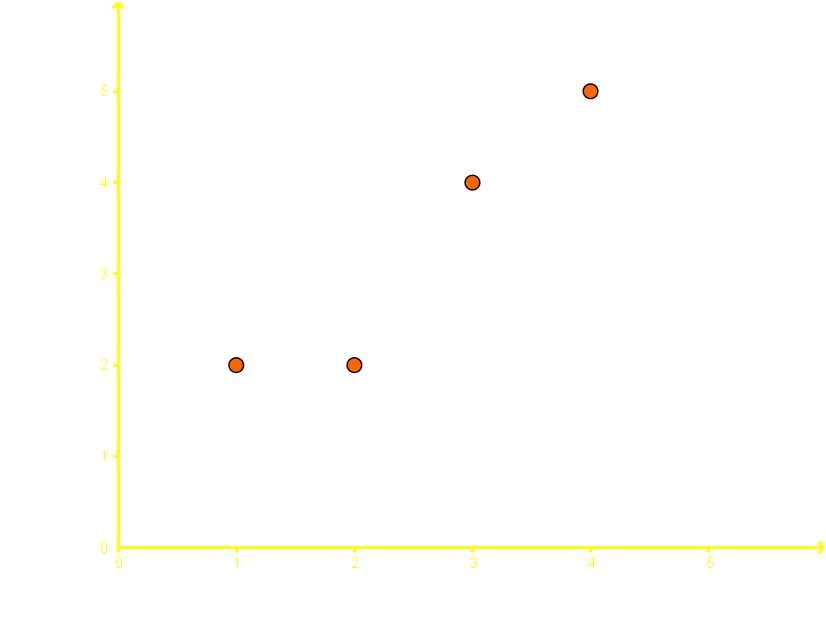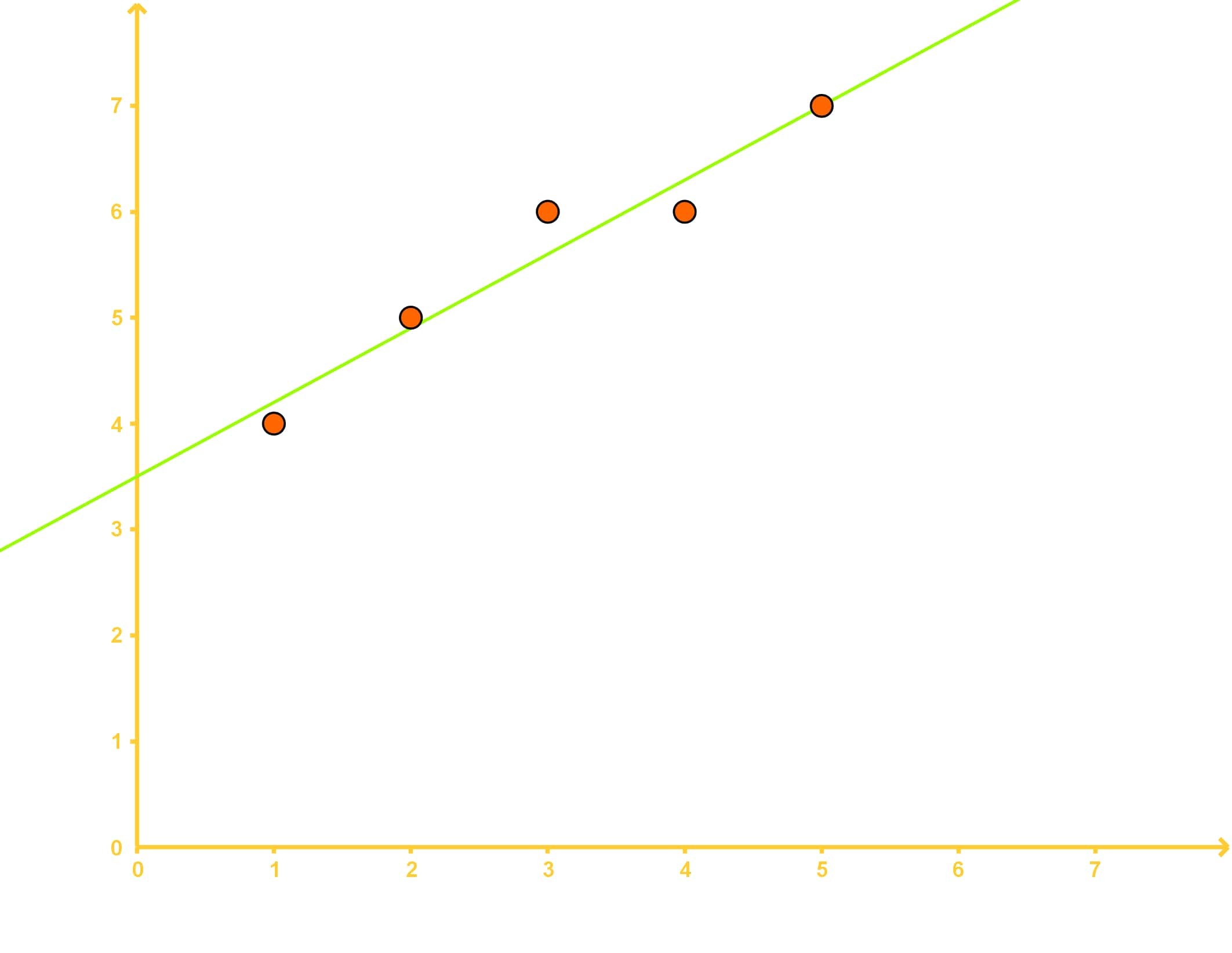Equation of the best fit line

Equation of the best fit line

Lessons

The best fit line has the equation: $y=ax+b$, where $a$ and $b$ are given as:
$a=\frac{n\sum xy-\sum x \sum y}{n\sum x^2-(\sum x)^2}$
$b=\overline{y}-a\overline{x}$
• Introduction

• Formula for the Best Fit Line
• What are Residuals?

• 1.
Determining the Equation for a Best Fit Line
Given the following bivariate data give the equation for the best fit line and plot it on the given graph.
 x y 1 2 2 2 3 4 4 5• 2.
Determining the Equation for a Best Fit Line using Calculator Commands
For the following bivariate data:
 x y 1 9 2 7 3 8 4 5 5 5 6 3 7 2

a)
Using a graphing calculator plot the points on a graph

b)
Still using your graphing calculator find the equation for the best fit line and plot it on the same graph

• 3.
Interpretation graphical Data
In Skyrim (a video game) I plotted what level I was when I killed my first 5 dragons. The graphical data is given below:
 # of dragons killed Corresponding level 1 Level 4 2 Level 5 3 Level 6 4 Level 6 5 Level 7a)
What is the sum of all the residuals squared?

b)
Using the data above extrapolate what my level will be when I kill my 8th dragon. Is this a good estimation? Why or why not?